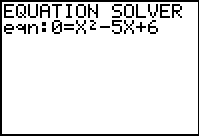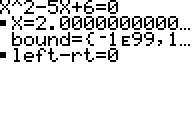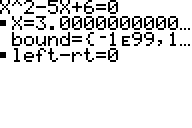# Knowledge Base

## Solution 11989: Solving a Quadratic Equation Using Numeric Solver on a TI-83 Family, TI-84 Plus Family or TI-Nspire™ Handheld in TI-84 Plus Mode.

### How can I solve a quadratic equation using Numeric Solver on a TI-83 family, TI-84 Plus family or TI-Nspire handheld in TI-84 Plus mode?

The numeric "Solver" feature is limited to solving for only one solution at a time. Because there are generally two solutions for a quadratic equation, two different guesses must be entered into the solver to find both solutions. After finding the first solution, enter a new initial guess or new bounds to look for the second solution.

Follow the steps below to solve the example equation: x^2=5x-6.

1) First, re-write the equation to make it equal to zero. The new equation would be: x^2-5x+6=0.
2) On the TI-84 Plus family or the TI-Nspire in TI-84 Plus mode press [MATH] [ALPHA] [B]. On the TI-83 Plus family press [MATH] .
3) If no equation is stored. the screen will display eqn:0=. If a previous equation was stored, press the up arrow until you get to the first screen.
4) Enter in x^2-5x+65) Press [ENTER] (the cursor will be blinking next to X=).
6) Enter a guess. Let's pick (-3) for example. The screen should have X=-3.
7) Press [ALPHA] [ENTER]. The answer x=2 or x=1.9999999 will be displayed depending on the calculator's mode setting.8) To find the second solution to the equation, repeat the procedure above and enter a different guess. For example, using X=5, the answer X=3 will be returned.Please see the TI-83 Plus Family and TI-84 Plus Family guidebooks for additional information.

TI-Nspire handheld in TI-84 Plus mode users may refer to the TI-84 Plus family guidebook.